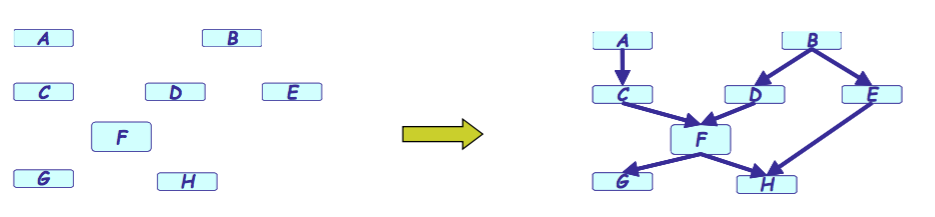# Probabilistic Graphical Models (1): Introduction

What is probabilistic graphical model (PGM)? Forget about some high-level and complicated sentences on textbook or wikipedia. From my perspective, it’s all about graphs, distributions and the connections between them.

There are three aspects to a graphical model:

• The graph
• A model of the data based on the graph
• The data itself

Actually, the data is generated by the underlying distribution and the model is the connection between the graph and the distribution. Here comes three fundamental issues:

• Representation: How to represent the three aspects mentioned above succinctly?
• Inference: How do I answser questions/queries according to my model and the given data? For example, the probability of a certain variable given some observations $P(X_i \vert \mathcal{D})$.
• Learning: What model is “right” for my data? We may want to “learn” the parameters of the model, or the model itself or even the topology of the graph from the data.

But, why we need to use PGM? Consider a multivariate distribution on $8$ binary-valued variables $X_1,\dots, X_8$. Such a distribution contains $2^8$ configurations and the specification of the joint distribution would require $2^8-1$ numbers. In pratical, quite a bit of these configurations are unnecessary. In this form, inference is also expensive as it would, in general, require summing over an exponential number of configurations of unobserved variables. Learning is also problematic in this case because huge amounts of data are needed to accurately learn probabilities, especially of rare events. A solution to this problem is to use conditional independencies. Conditional independencies are statements of the form “$X$ is independent of $Y$ given $Z$” or $X \perp Y \vert Z$. If all the variables are independent, we can write:

This representation reduces parameter requirement from exponential to linear in the number of variables. But this representation is not rich enough to capture all possibilities. PGM try to capture middle ground by using conditional independencies known from the domain. Graph representation captures these conditional independencies compactly.So, what is a PGM after all? It’s a smart way to specify exponentially-large probability distributions without paying an exponential cost, and at the same time endow the distributions with structured semantics. More formally, it refers to a family of distributions on a set of random variables that are compatible with all the probabiliistic independence propositions encoded by a graph that connects these variables.